# Math Worksheets Land

Math Worksheets For All Ages

# Math Worksheets Land

Math Worksheets For All Ages

# Basic Operations with Decimals Worksheets

When you are working on calculations of just about any math operation that includes a decimal it is not that tricky, you just need to account for the decimal points throughout the process. Addition and subtraction can be processed pretty much in the same manner, you just need to line up all the place values. You may need to borrow and carry values, just like you would normally do with those operations. When we multiply, you can simply remove the decimals and process the problem as if they were not present. You just need to count how many places you need account for and place them back in at the end. Division is the tricky operation because it is not super straight forward. Take a look at how to process all these operations on the lesson or tutorial found below. These worksheets will help students become comfortable processing these operations.

### Aligned Standard: Grade 6 Numbers - 6.NS.B.3

• Answer Keys - These are for all the unlocked materials above.

### Homework Sheets

Sums, difference, products, and quotients... We cover it all in here with decimals.

• Homework 1 - Add the numbers as we would add whole numbers, but make sure to drop the decimal point.
• Homework 2 - Multiply as we would multiply whole numbers without a decimal point.
• Homework 3 - Divide until there is no remainder.

### Practice Worksheets

I got all the operations into every sheet and made the font pretty big for practice.

• Practice 1 - Subtract the numbers just as we normally would subtract whole numbers. Make sure to leave room for the decimal point.
• Practice 2 - All four operations are explored here.
• Practice 3 - Count the total number of decimal places in the factors.

### Math Skill Quizzes

I tried to space everything out as best that I could, but it is hard to contain everything to one page.

• Quiz 1 - Don't mind the decimal points. They are just decoration.
• Quiz 2 - We cover all the different operations with decimals. See how well you do with these problems.
• Quiz 3 - All focused on adding and subtracting.

### How to Add, Subtract, Multiply, and Divide Decimal Values

These two operations are very similar in the approach that you take when setting up these calculations. Finding the sums and differences of decimal values is all about staying organized and realizing where the decimal point lies throughout the entire process.

Lining up the Decimals - This means that we perfectly align one decimal value above the other. I would get in the habit of place the value that has more decimal values on top, you will see why in a minute.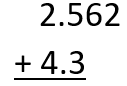Pad Empty Spaces with Zeroes - As we can see the hundredths and thousandths place of the bottom addend are not existence. If there is nothing present that is the same thing as saying zero. You can pad those empty spaces with zeroes. Here is what it looks like: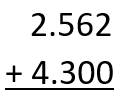Add Columns from Right to Left - We now add each column starting far right and moving to the left, just like normal addition. The only difference is we need to keep track of the decimal point.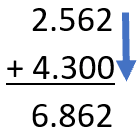Subtraction- We will use the example of subtracting 5.827 - 3.61

Lining up the Decimals - Just like addition, it helps to line these problems vertically. We place the minuend over the subtrahend to find the difference. Again, we make sure that our decimal points line up.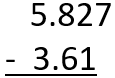Pad Empty Spaces with Zeroes - We can see that our subtrahend (3.61) is missing a thousandths place so we can pad it with a zero at that space.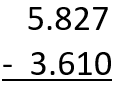Subtract Columns from Right to Left - We find the difference of each value moving from the right column to the left.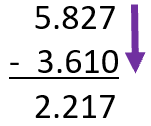Multiplication- Let us take an example of 4.21 x 4.1 and solve it individually.

Multiplication becomes very easy when you learn its method. However, when you learn how to multiply whole numbers, multiplying and dividing decimals become easier as well. Let us consider examples of both decimal multiplication and division one by one.

<
p>First, you multiply the number like there is no decimal point there.

421 x 41 = 17261

Now, there are two decimal places in 4.21 and only one in 4.1. So, the total decimal places are 3. Then, the answer becomes 17.261.

Division - Dividing decimal numbers is very similar to the multiplying them. You just divide the numbers by removing the decimals. At this point we should have a good idea on how to do this.

For example, you have to divide 46 ÷ 2.3.

As we have done before we remove the decimal point and count how many place values were present. In this example, we make 2.3 into 23. However, you see that there is no decimal in 46, but since you placed the point after one decimal place. In the case of 46, a zero will be added to make it 460. Now, you just divide 460 by 23 and we will get an answer of 20.

Unlock all the answers, worksheets, homework, tests and more!
Save Tons of Time! Make My Life Easier Now

## Thanks and Don't Forget To Tell Your Friends!

I would appreciate everyone letting me know if you find any errors. I'm getting a little older these days and my eyes are going. Please contact me, to let me know. I'll fix it ASAP.